# KEYS R workshopThis is a short 1 hour and 45 minutes introduction to R and the tidyverse prepared and delivered by Kelsey Gonzalez for BIO5 Institute’s KEYS (Keep Engaging Youth in Science) Research Internship Program on June 10, 2020.

A PDF of this lesson is available here

This lesson is largely adapted from Software and Data Carpentry materials by Kelsey Gonzalez for use with the Penguins dataset.

# Getting set up

R works by having a base programming language that can be added on to with community-created open-source extensions called packages. We’re going to first install two packages that we will work with today.

``````install.packages("tidyverse")
install.packages("palmerpenguins")``````
``````library(tidyverse)
library(palmerpenguins)``````

## What is an R package?

An R package is a complete unit for sharing code with others. Each R package contains the code for a set of R functions, the documentation (or description) for each of the functions, as well as a practice dataset to learn the functions on.

Generally, each R package is built with a specific task in mind. For instance, the package `dplyr` provides easy tools for the most common data manipulation tasks. It is built to work directly with data frames, with many common tasks optimized by being written in a compiled language (C++) (not all R packages are written in R!).

But there are also packages available for a wide range of tasks including building plots (`ggplot2`, which we’ll see later), downloading data from the NCBI database, or performing statistical analysis on your data set. Many packages such as these are housed on, and downloadable from, the Comprehensive R Archive Network (CRAN) using `install.packages`. This function makes the package accessible by your R installation with the command `library()`, as you did with `tidyverse` earlier.

To easily access the documentation for a package within R or RStudio, use `help(package = "package_name")`.

We only have a short lesson today, so I won’t get into the specifics on what we’re doing here. Just know we loaded a package called penguins above which contained a dataset called penguins. By calling `data()`, we’re asking r to load a dataset that is in the package. `R` also can read csv files, excel files, and many other data types.

``data(penguins)``

# Data Wrangling & visualization:

questions:

• How can I select specific rows and/or columns from a data frame?
• How can I combine multiple commands into a single command?
• How can create new columns or remove existing columns from a data frame?
• How can I reformat a dataframe to meet my needs?

objectives:

• Describe the purpose of an R package and the `dplyr` and `tidyr` packages.
• Select certain columns in a data frame with the `dplyr` function `select`.
• Select certain rows in a data frame according to filtering conditions with the `dplyr` function `filter`.
• Link the output of one `dplyr` function to the input of another function with the ‘pipe’ operator `%>%`.
• Add new columns to a data frame that are functions of existing columns with `mutate`.
• Use the split-apply-combine concept for data analysis.
• Use `summarize`, `group_by`, and `count` to split a data frame into groups of observations, apply a summary statistics for each group, and then combine the results.
• Describe the concept of a wide and a long table format and for which purpose those formats are useful.
• Describe what key-value pairs are.
• Reshape a data frame from long to wide format and back with the `spread` and `gather` commands from the `tidyr` package.
• Export a data frame to a csv file.

keypoints:

• Use the `dplyr` package to manipulate dataframes.
• Use `select()` to choose variables from a dataframe.
• Use `filter()` to choose data based on values.
• Use `group_by()` and `summarize()` to work with subsets of data.
• Use `mutate()` to create new variables.
• Use the `tidyr` package to change the layout of dataframes.

## Data Manipulation using `dplyr`

`dplyr` is a package for making tabular data manipulation easier by using a limited set of functions that can be combined to extract and summarize insights from your data.

Similarly to `readr`, `dplyr` and `tidyr` are also part of the tidyverse. These packages were loaded in R’s memory when we called `library(tidyverse)` earlier.

To learn more about `dplyr` after the workshop, you may want to check out this handy data transformation with `dplyr` cheatsheet.

We’re going to learn some of the most common `dplyr` functions:

• `select()`: subset columns
• `filter()`: subset rows on conditions
• `mutate()`: create new columns by using information from other columns
• `group_by()` and `summarize()`: create summary statistics on grouped data
• `arrange()`: sort results

## Explore our data

Let’s explore our data first. There’s 7 variables or columns that we have to work with.

• `species`: penguin species (Chinstrap, Adélie, or Gentoo)
• `bill_length_mm`: bill length (mm)
• `bill_depth_mm`: bill depth (mm)
• `flipper_length_mm`: flipper length (mm)
• `body_mass_g`: body mass (g)
• `island`: island name (Dream, Torgersen, or Biscoe) in the Palmer Archipelago (Antarctica)
• `sex`: penguin sex

Here’s what the three penguins species look like in real life:We have a few options to preview our dataframe. My favorite is `glimpse()`

``glimpse(penguins)``

You can also `View()` the data, which opens the data in a R Studio viewer pane. Note the capital V.

``View(penguins)``

## Selecting columns and filtering rows

To select columns of a data frame, use `select()`. The first argument to this function is the data frame (`penguins`), and the subsequent arguments are the columns to keep.

``select(penguins, species, body_mass_g, sex)``

To choose rows based on a specific criteria, use `filter()`:

``filter(penguins, island == "Biscoe")``

The filter function works with most boolean operators, namely:

Operator Description
`<` less than
`<=` less than or equal to
`>` greater than
`>=` greater than or equal to
`==` exactly equal to
`!=` not equal to
`!x` Not x
`x | y` x OR y
`x & y` x AND y
`isTRUE(x)` test if X is TRUE

## Pipes

What if you want to select and filter at the same time? There are three ways to do this: use intermediate steps, nested functions, or pipes.

With intermediate steps, you create a temporary data frame and use that as input to the next function, like this:

``````penguins_biscoe <- filter(penguins, island == "Biscoe")
penguins_biscoe <- select(penguins_biscoe, species, body_mass_g, sex)``````

This is readable, but can clutter up your workspace with lots of objects that you have to name individually. With multiple steps, that can be hard to keep track of.

You can also nest functions (i.e. one function inside of another), like this:

``penguins_Biscoe <- select(filter(penguins, island == "Biscoe"),  species, body_mass_g, sex)``

This is handy, but can be difficult to read if too many functions are nested, as R evaluates the expression from the inside out (in this case, filtering, then selecting).

The last option, pipes, are a powerful addition to R. Pipes let you take the output of one function and send it directly to the next, which is useful when you need to do many things to the same dataset. Pipes in R look like `%>%` and are made available via the `magrittr` package, installed automatically with `dplyr`. If you use RStudio, you can type the pipe with Ctrl + Shift + M if you have a PC or Cmd + Shift + M if you have a Mac.

``````penguins %>%
filter(island == "Biscoe") %>%
select(species, body_mass_g, sex)``````
``````## # A tibble: 168 x 3
##    species body_mass_g sex
##    <fct>         <int> <fct>
## # … with 158 more rows``````

In the above code, we use the pipe to send the `penguins` dataset first through `filter()` to keep rows where `island` is “Biscoe”, then through `select()` to keep only the `species`, `body_mass_g`,and `sex` columns. Since `%>%` takes the object on its left and passes it as the first argument to the function on its right, we don’t need to explicitly include the data frame as an argument to the `filter()` and `select()` functions any more.

Some may find it helpful to read the pipe like the word “then”. For instance, in the above example, we take the data frame `penguins`, then we `filter` for rows with `island == "Biscoe"`, then we `select` columns `species`, `body_mass_g`,and `sex`. The `dplyr` functions by themselves are somewhat simple, but by combining them into linear workflows with the pipe, we can accomplish more complex manipulations of data frames.

If we want to create a new object with this smaller version of the data, we can assign it a new name:

``````penguins_biscoe <- penguins %>%
filter(island == "Biscoe") %>%
select(species, body_mass_g, sex)

penguins_biscoe``````
``````## # A tibble: 168 x 3
##    species body_mass_g sex
##    <fct>         <int> <fct>
## # … with 158 more rows``````

Note that the final data frame (`penguins_biscoe`) is the leftmost part of this expression becuase it is receiving an assignment.

## Exercise

Using pipes, subset the `penguins` data to include all species EXCEPT Adelie and retain the species column in addition to those relating to their bill. ## Solution

``````penguins %>%
select(bill_length_mm, bill_depth_mm)``````

### Mutate

Frequently you’ll want to create new columns based on the values in existing columns, for example to do unit conversions, or to find the ratio of values in two columns. For this we’ll use `mutate()`.

We might be interested in the flipper length of penguins in cm instead of milimeters:

``````penguins %>%
mutate(flipper_length_cm = flipper_length_mm / 10)``````
``````## # A tibble: 344 x 9
##    species island bill_length_mm bill_depth_mm flipper_length_… body_mass_g
##    <fct>   <fct>           <dbl>         <dbl>            <int>       <int>
##  1 Adelie  Torge…           39.1          18.7              181        3750
##  2 Adelie  Torge…           39.5          17.4              186        3800
##  3 Adelie  Torge…           40.3          18                195        3250
##  4 Adelie  Torge…           NA            NA                 NA          NA
##  5 Adelie  Torge…           36.7          19.3              193        3450
##  6 Adelie  Torge…           39.3          20.6              190        3650
##  7 Adelie  Torge…           38.9          17.8              181        3625
##  8 Adelie  Torge…           39.2          19.6              195        4675
##  9 Adelie  Torge…           34.1          18.1              193        3475
## 10 Adelie  Torge…           42            20.2              190        4250
## # … with 334 more rows, and 3 more variables: sex <fct>, year <int>,
## #   flipper_length_cm <dbl>``````

We may be interested in investigating whether being a member of an irrigation association had any effect on the ratio of household members to rooms. To look at this relationship, we will first remove data from our dataset where the respondent didn’t answer the question of whether they were a member of an irrigation association. These cases are recorded as “NULL” in the dataset.

## Exercise

Create a new data frame from the `penguins` data that meets the following criteria: contains only the `species` column and a new column called `body_mass_kg` containing a transformed body_mass_g. Only the rows where `body_mass_kg` is greater than 4 should be shown in the final data frame. How many rows do you have?

Hint: think about how the commands should be ordered to produce this data frame!

## Solution

``````penguins_large <- penguins %>%
mutate(body_mass_kg = body_mass_g / 1000) %>%
filter(body_mass_kg > 4) %>%
select(species, body_mass_kg)``````

### Split-apply-combine data analysis and the summarize() function

Many data analysis tasks can be approached using the split-apply-combine paradigm: split the data into groups, apply some analysis to each group, and then combine the results. `dplyr` makes this very easy through the use of the `group_by()` function.

#### The `summarize()` function

`group_by()` is often used together with `summarize()`, which collapses each group into a single-row summary of that group. `group_by()` takes as arguments the column names that contain the categorical variables for which you want to calculate the summary statistics. So to compute the average household size by island:

``````penguins %>%
group_by(island) %>%
summarize(mean_flipper_length_mm = mean(flipper_length_mm))``````
``````## # A tibble: 3 x 2
##   island    mean_flipper_length_mm
##   <fct>                      <dbl>
## 1 Biscoe                       NA
## 2 Dream                       193.
## 3 Torgersen                    NA``````

You may also have noticed that the output has a lot of `NA`! When `R` does calculations with missing data, it (correctly) doesn’t know how to evaluate them and forces the result to `NA`. to solve this, we need to add in a special option to tell R that we want to ignore the missing values.

Use the `?` function on `mean()` to figure out what this option is.

``````penguins %>%
group_by(island) %>%
summarize(mean_flipper_length_mm = mean(flipper_length_mm, na.rm = TRUE))``````
``````## # A tibble: 3 x 2
##   island    mean_flipper_length_mm
##   <fct>                      <dbl>
## 1 Biscoe                      210.
## 2 Dream                       193.
## 3 Torgersen                   191.``````

You can also group by multiple columns:

``````penguins %>%
group_by(island, species) %>%
summarize(mean_flipper_length_mm = mean(flipper_length_mm, na.rm = TRUE))``````
``````## # A tibble: 5 x 3
## # Groups:   island 
##   island    species   mean_flipper_length_mm
##   <fct>     <fct>                      <dbl>
## 2 Biscoe    Gentoo                      217.
## 4 Dream     Chinstrap                   196.

I expected to get 9 rows because we have 3 islands and 3 species of penguin. What do you think is going on here?

Once the data are grouped, you can also summarize multiple variables at the same time (and not necessarily on the same variable). For instance, we could add a column indicating the minimum and maximum flipper length for each island for each species:

``````penguins %>%
group_by(island, species) %>%
summarize(mean_flipper_length_mm = mean(flipper_length_mm, na.rm = TRUE),
min_flipper_length_mm = min(flipper_length_mm, na.rm = TRUE),
max_flipper_length_mm = max(flipper_length_mm, na.rm = TRUE))``````
``````## # A tibble: 5 x 5
## # Groups:   island 
##   island   species   mean_flipper_lengt… min_flipper_length… max_flipper_length…
##   <fct>    <fct>                   <dbl>               <int>               <int>
## 1 Biscoe   Adelie                   189.                 172                 203
## 2 Biscoe   Gentoo                   217.                 203                 231
## 3 Dream    Adelie                   190.                 178                 208
## 4 Dream    Chinstrap                196.                 178                 212
## 5 Torgers… Adelie                   191.                 176                 210``````

It is sometimes useful to rearrange the result of a query to inspect the values. For instance, we can sort on `mean_flipper_length_mm` to put the group with the shortest flippers first:

``````penguins %>%
group_by(island, species) %>%
summarize(mean_flipper_length_mm = mean(flipper_length_mm, na.rm = TRUE),
min_flipper_length_mm = min(flipper_length_mm, na.rm = TRUE),
max_flipper_length_mm = max(flipper_length_mm, na.rm = TRUE)) %>%
arrange(mean_flipper_length_mm)``````
``````## # A tibble: 5 x 5
## # Groups:   island 
##   island   species   mean_flipper_lengt… min_flipper_length… max_flipper_length…
##   <fct>    <fct>                   <dbl>               <int>               <int>
## 1 Biscoe   Adelie                   189.                 172                 203
## 2 Dream    Adelie                   190.                 178                 208
## 3 Torgers… Adelie                   191.                 176                 210
## 4 Dream    Chinstrap                196.                 178                 212
## 5 Biscoe   Gentoo                   217.                 203                 231``````

To sort in descending order, we need to add the `desc()` function.

``````penguins %>%
group_by(island, species) %>%
summarize(mean_flipper_length_mm = mean(flipper_length_mm, na.rm = TRUE),
min_flipper_length_mm = min(flipper_length_mm, na.rm = TRUE),
max_flipper_length_mm = max(flipper_length_mm, na.rm = TRUE)) %>%
arrange(desc(mean_flipper_length_mm))``````
``````## # A tibble: 5 x 5
## # Groups:   island 
##   island   species   mean_flipper_lengt… min_flipper_length… max_flipper_length…
##   <fct>    <fct>                   <dbl>               <int>               <int>
## 1 Biscoe   Gentoo                   217.                 203                 231
## 2 Dream    Chinstrap                196.                 178                 212
## 3 Torgers… Adelie                   191.                 176                 210
## 4 Dream    Adelie                   190.                 178                 208
## 5 Biscoe   Adelie                   189.                 172                 203``````

## Exercise:

Use `group_by()` and `summarize()` to find the mean bill_length and bill_depth each species.

## Solution

``````penguins %>%
group_by(species) %>%
summarize(
mean_bill_length = mean(bill_length_mm, na.rm = TRUE),
mean_bill_depth = mean(bill_depth_mm, na.rm = TRUE)
)``````

# Data Visualization

questions:

• What are the components of a ggplot?
• How do I create scatterplots, boxplots, and barplots?
• How can I change the aesthetics (ex. colour, transparency) of my plot?
• How can I create multiple plots at once?

objectives:

• Produce scatter plots, boxplots, and time series plots using ggplot.
• Set universal plot settings.
• Describe what faceting is and apply faceting in ggplot.
• Modify the aesthetics of an existing ggplot plot (including axis labels and color).
• Build complex and customized plots from data in a data frame.

keypoints:

• `ggplot2` is a flexible and useful tool for creating plots in R.
• The data set and coordinate system can be defined using the `ggplot` function.
• Additional layers, including geoms, are added using the `+` operator.
• Boxplots are useful for visualizing the distribution of a continuous variable.
• Barplot are useful for visualizing categorical data.
• Faceting allows you to generate multiple plots based on a categorical variable.

## Plotting with `ggplot2`

`ggplot2` is a plotting package that makes it simple to create complex plots from data stored in a data frame. It provides a programmatic interface for specifying what variables to plot, how they are displayed, and general visual properties. Therefore, we only need minimal changes if the underlying data change or if we decide to change from a bar plot to a scatterplot. This helps in creating publication quality plots with minimal amounts of adjustments and tweaking.

ggplot graphics are built step by step by adding new elements. Adding layers in this fashion allows for extensive flexibility and customization of plots.

To learn more about `ggplot2` after the workshop, you may want to check out this handy data transformation with `ggplot2` cheatsheet.

To build a ggplot, we will use the following basic template that can be used for different types of plots:

``ggplot(data = <DATA>, mapping = aes(<MAPPINGS>)) +  <GEOM_FUNCTION>()``
• use the `ggplot()` function and bind the plot to a specific data frame using the `data` argument
``ggplot(data = penguins)``
• define a mapping (using the aesthetic (`aes`) function), by selecting the variables to be plotted and specifying how to present them in the graph, e.g. as x/y positions or characteristics such as size, shape, color, etc.
``ggplot(data = penguins, aes(x = flipper_length_mm, y = body_mass_g)``
• add ‘geoms’ – graphical representations of the data in the plot (points, lines, bars). `ggplot2` offers many different geoms; we will use some common ones today, including:

• `geom_point()` for scatter plots, dot plots, etc.

• `geom_boxplot()` for, well, boxplots!

• `geom_line()` for trend lines, time series, etc.

To add a geom to the plot use the `+` operator. Because we have two continuous variables, let’s use `geom_point()` first:

``````ggplot(data = penguins, aes(x = flipper_length_mm, y = body_mass_g)) +
geom_point()``````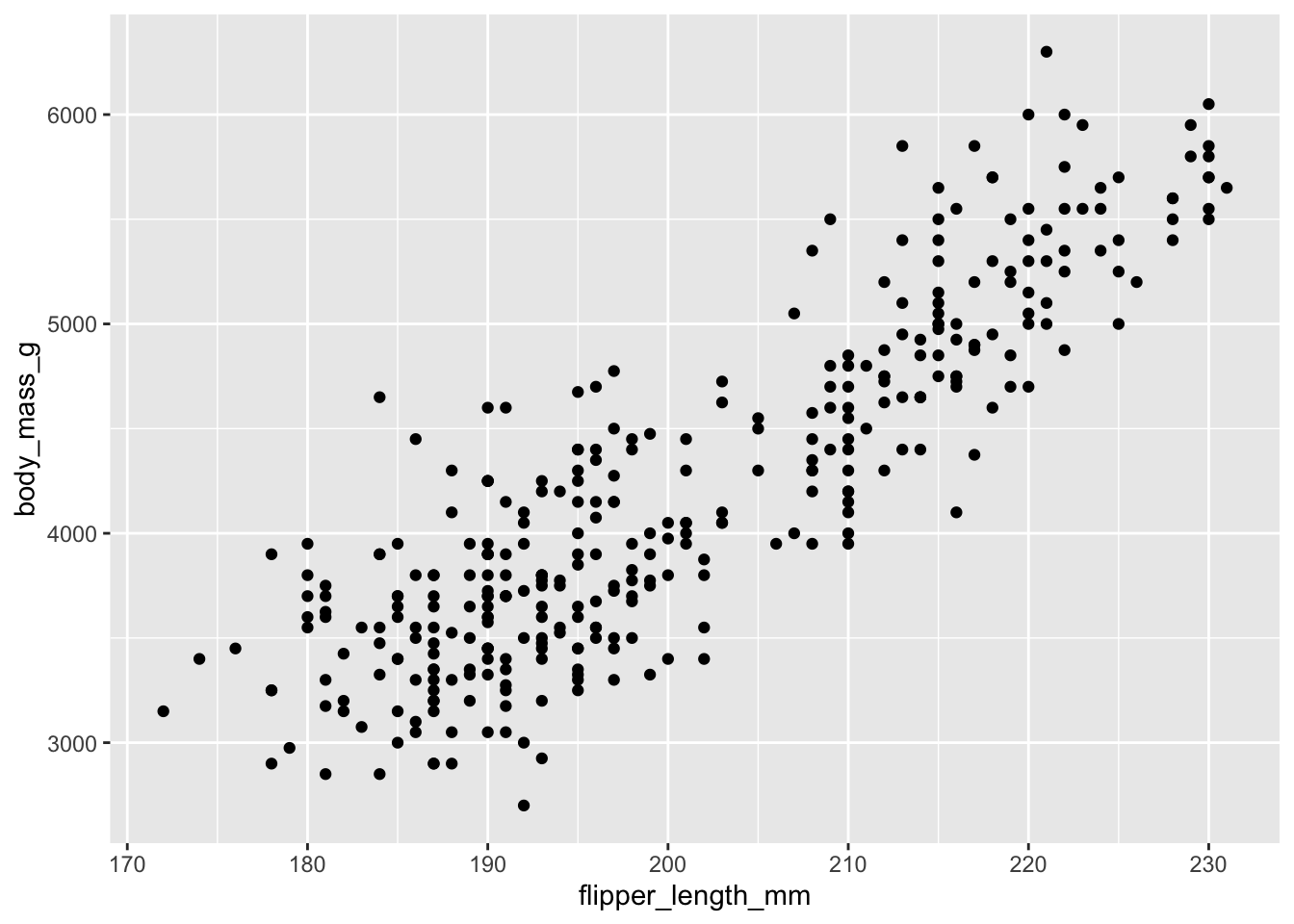The `+` in the `ggplot2` package is particularly useful because it allows you to modify existing `ggplot` objects. This means you can easily set up plot templates and conveniently explore different types of plots, so the above plot can also be generated with code like this:

``````# Assign plot to a variable
penguins_plot <- ggplot(data = penguins, aes(x = flipper_length_mm, y = body_mass_g))

# Draw the plot
penguins_plot +
geom_point()``````
• Anything you put in the `ggplot()` function can be seen by any geom layers that you add (i.e., these are universal plot settings). This includes the x- and y-axis mapping you set up in `aes()`.
• You can also specify mappings for a given geom independently of the mapping defined globally in the `ggplot()` function.
• The `+` sign used to add new layers must be placed at the end of the line containing the previous layer. If, instead, the `+` sign is added at the beginning of the line containing the new layer, `ggplot2` will not add the new layer and will return an error message.

Building plots with `ggplot2` is typically an iterative process. We start by defining the dataset we’ll use, lay out the axes, and choose a geom:

``````ggplot(data = penguins, aes(x = flipper_length_mm, y = body_mass_g)) +
geom_point()``````Then, we start modifying this plot to extract more information from it. For instance, we can add transparency (`alpha`) to avoid overplotting:

``````ggplot(data = penguins, aes(x = flipper_length_mm, y = body_mass_g)) +
geom_point(alpha = 0.5)``````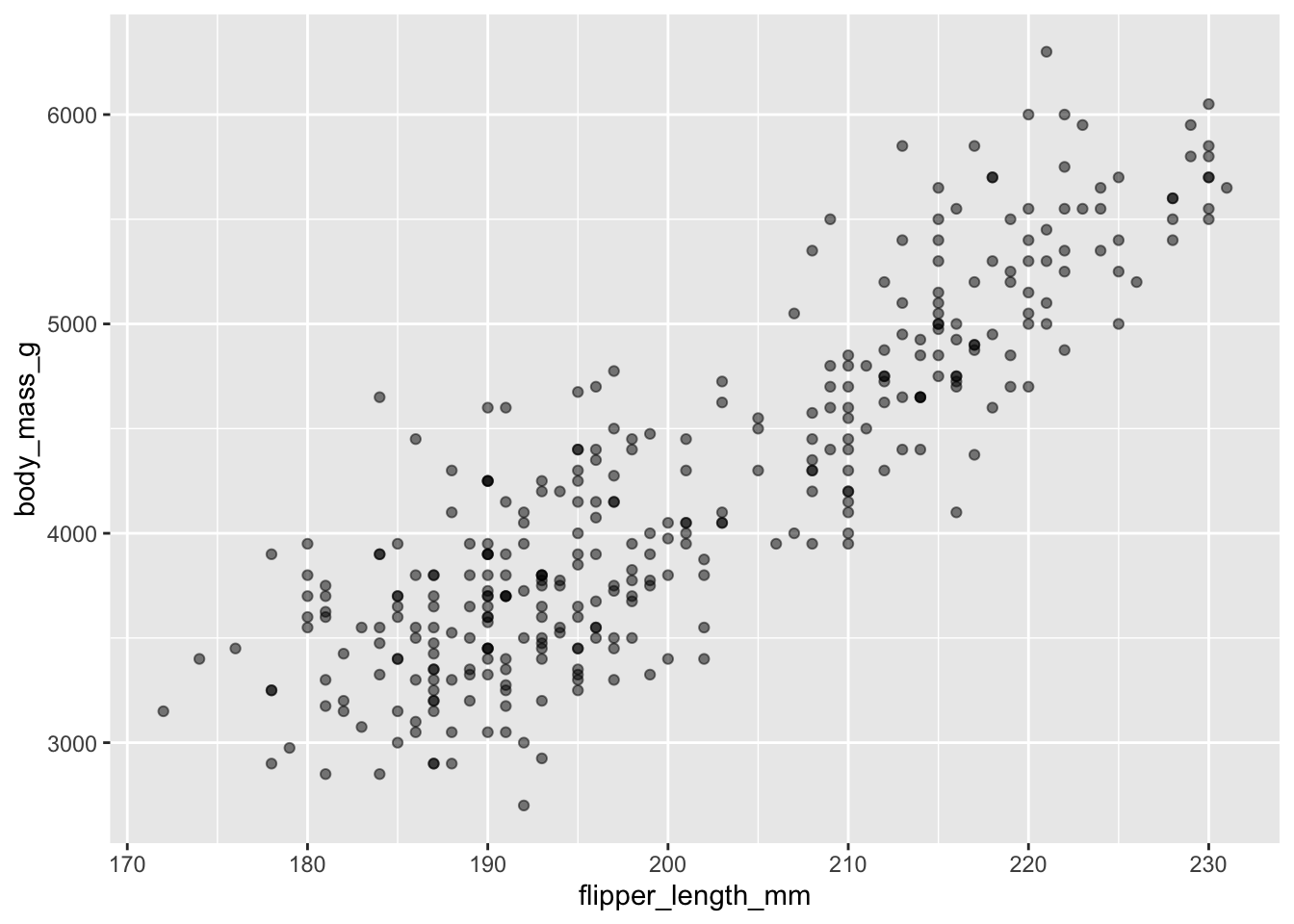That only helped a little bit with the overplotting problem. We can also introduce a little bit of randomness into the position of our points using the `geom_jitter()` function.

``````ggplot(data = penguins, aes(x = flipper_length_mm, y = body_mass_g)) +
geom_jitter(alpha = 0.5)``````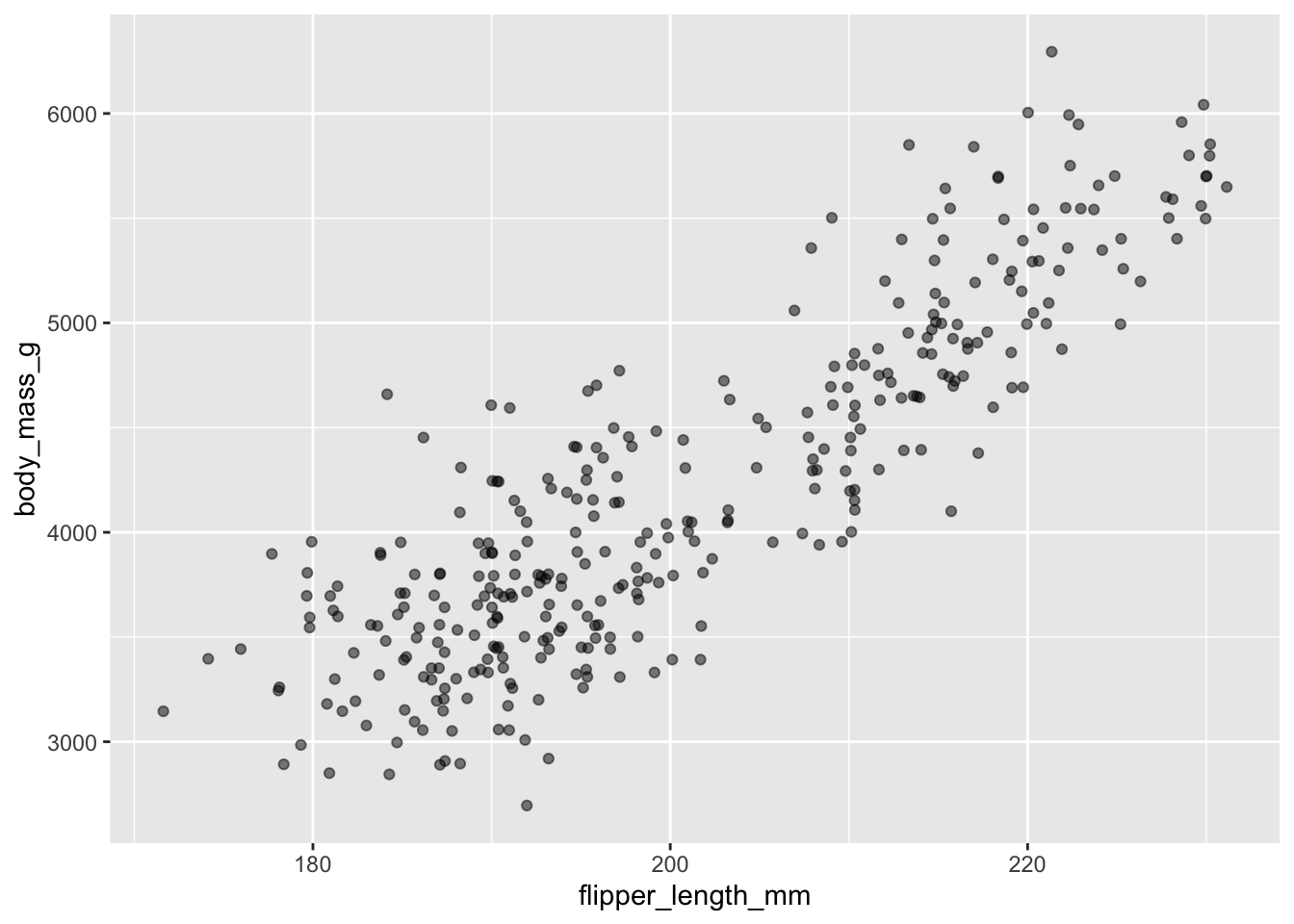We can also add colors for all the points:

``````ggplot(data = penguins, aes(x = flipper_length_mm, y = body_mass_g)) +
geom_jitter(alpha = 0.5, color = "blue")``````Or to color each species in the plot differently, you could use a vector as an input to the argument `color`. Because we are now mapping features of the data to a color, instead of setting one color for all points, the color now needs to be set inside a call to the `aes` function. `ggplot2` will provide a different color corresponding to different values in the vector. We set the value of `alpha` outside of the `aes` function call because we are using the same value for all points. Here is an example where we color by `island`:

``````ggplot(data = penguins, aes(x = flipper_length_mm, y = body_mass_g)) +
geom_jitter(aes(color = species), alpha = 0.5)``````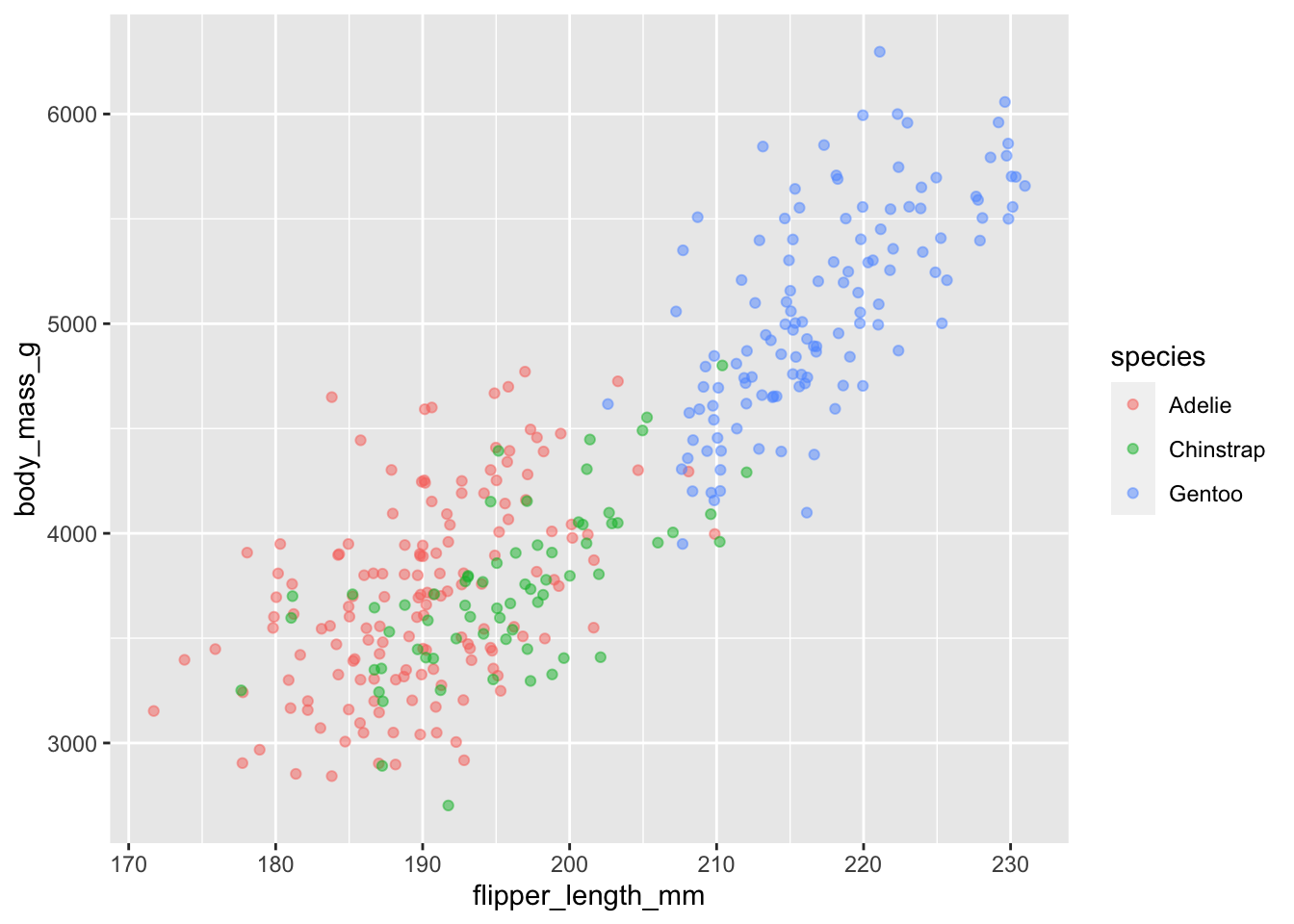There appears to be a positive trend between flipper length and body massof penguins. This trend does not appear to be different by species, but we can see that Chinstrap and Adelie penguins have shorter flippers and small body mass than Gentoo.

By default, the axes labels on a plot are determined by the name of the variable being plotted. However, `ggplot2` offers lots of customization options, like specifying the axes labels, and adding a title to the plot with relatively few lines of code. We will add more informative x and y axis labels to our plot of proportion of house type by island and also add a title.

``````ggplot(data = penguins, aes(x = flipper_length_mm, y = body_mass_g)) +
geom_jitter(aes(color = species), alpha = 0.5) +
labs(title="Body Mass by Flipper Length",
x="Flipper Length (mm)",
y="Body Mass (g)")``````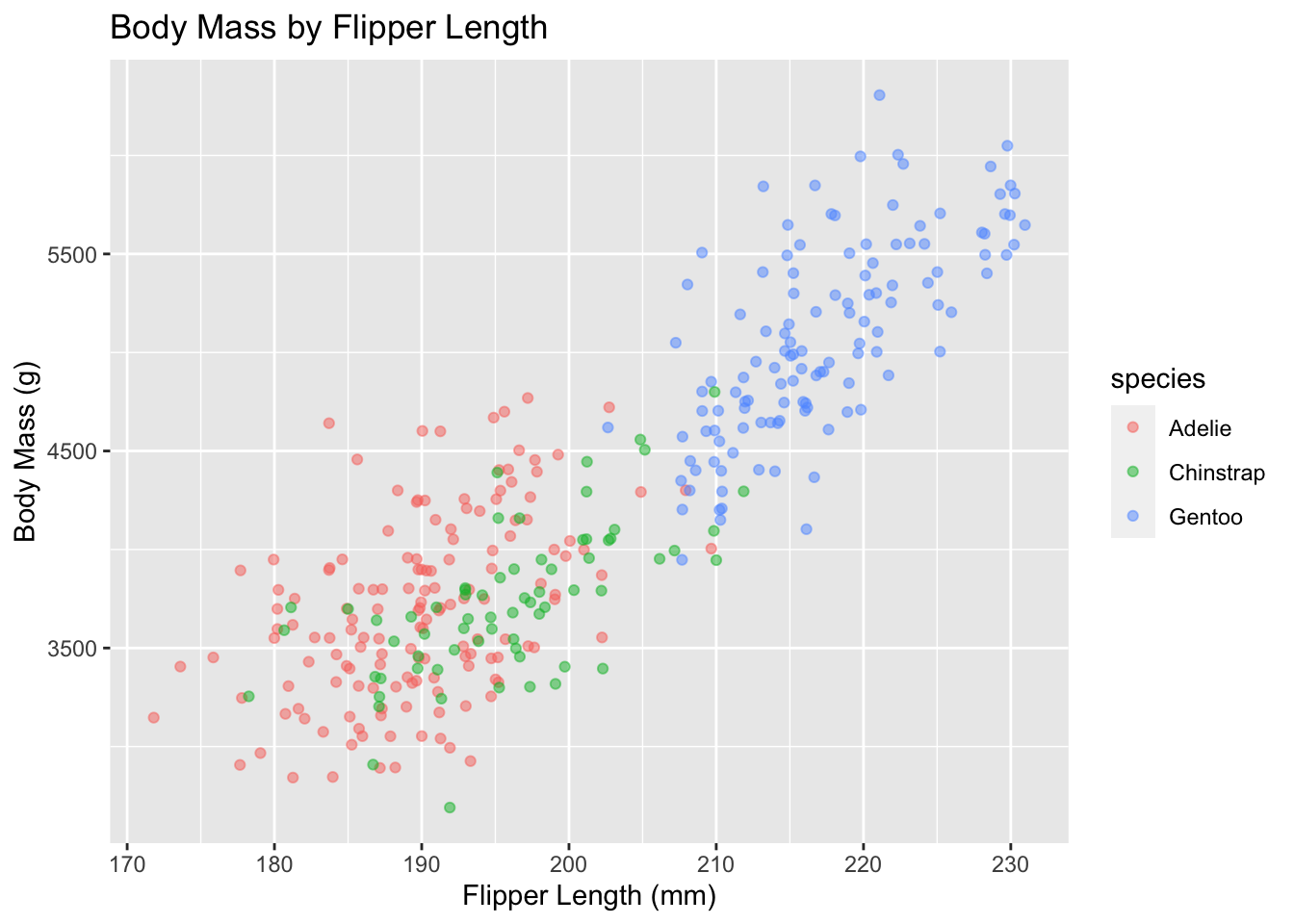## Exercise

Use what you just learned to create a scatter plot of `bill_length_mm` by `species` with the `sex` showing in different colors. Is this a good way to show this type of data?

## Solution

``````ggplot(data = penguins, aes(x = species, y = bill_length_mm)) +
geom_jitter(aes(color = sex))``````

This is not a good way to show this type of data because it is difficult to distinguish between islands.

## Boxplot

We can use boxplots to visualize the distribution of rooms for each wall type:

``````ggplot(data = penguins, aes(x = species, y = bill_length_mm)) +
geom_boxplot()``````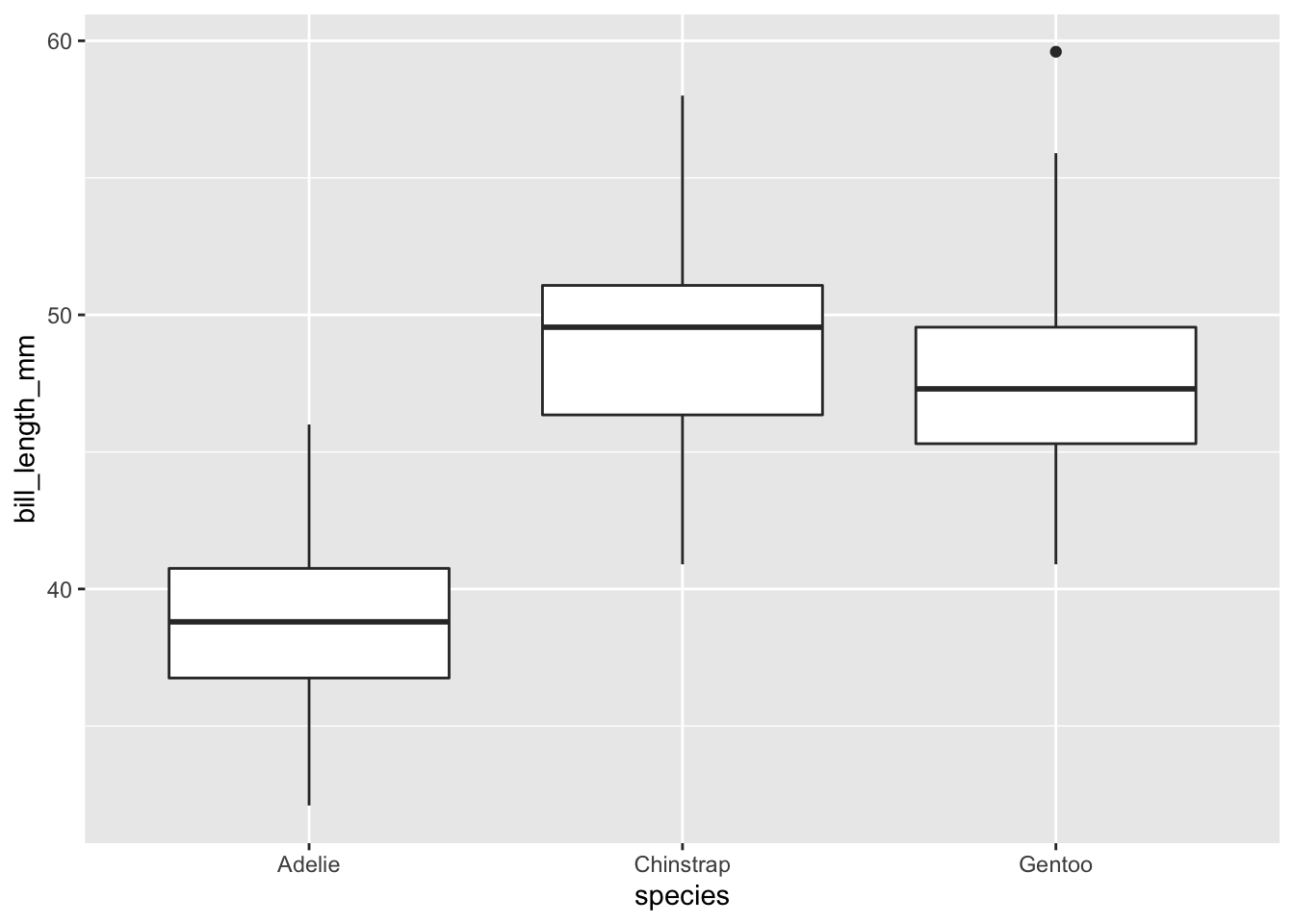By adding points to a boxplot, we can have a better idea of the number of measurements and of their distribution:

``````ggplot(data = penguins, aes(x = species, y = bill_length_mm)) +
geom_boxplot(alpha = 0) +
geom_jitter(alpha = 0.5, color = "tomato")``````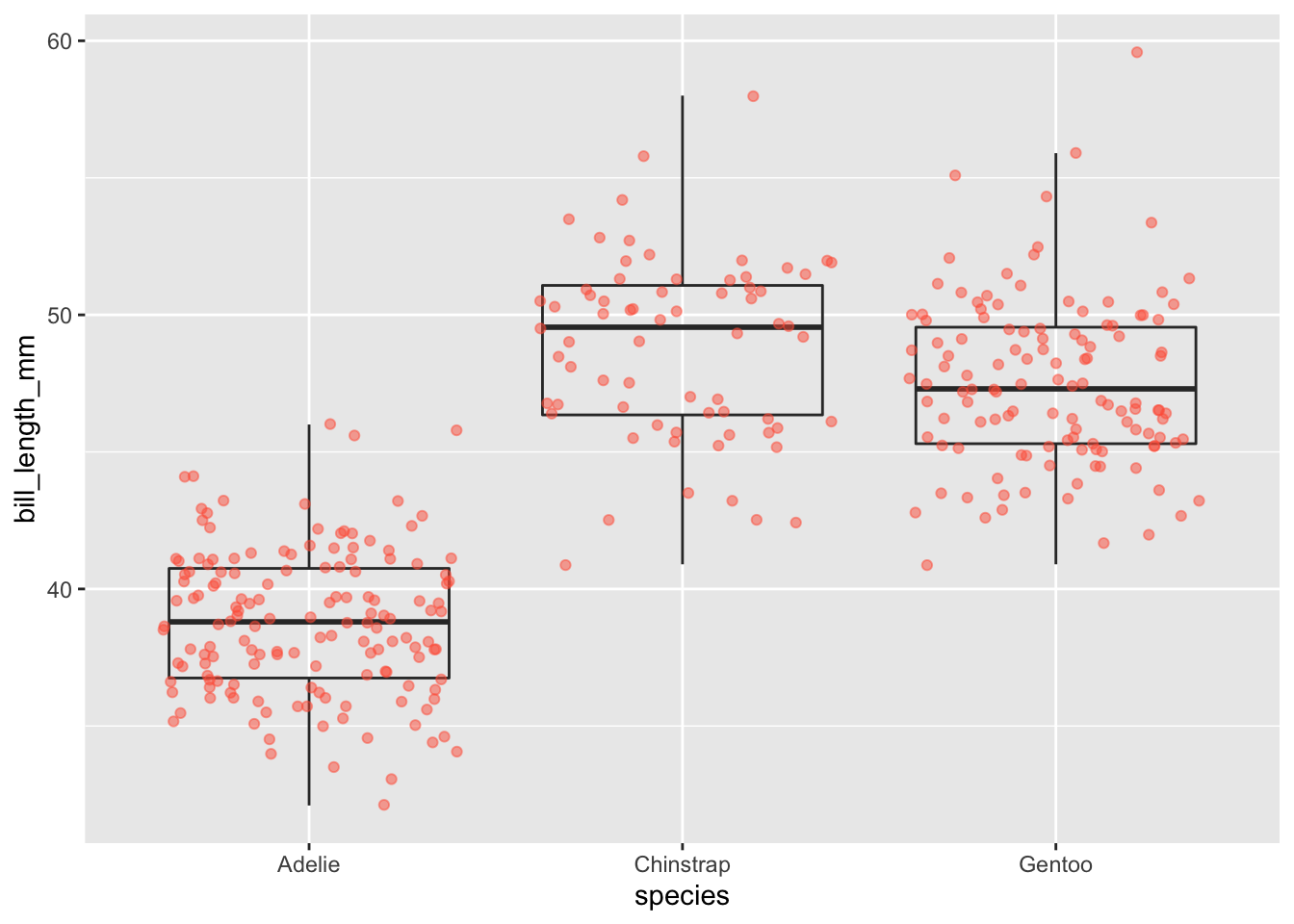We can see that Adelie penguins tend to have shorter bills than other species.

Notice how the boxplot layer is behind the jitter layer? What do you need to change in the code to put the boxplot in front of the points such that it’s not covered?

## Exercise

Boxplots are useful summaries, but hide the shape of the distribution. For example, if the distribution is bimodal, we would not see it in a boxplot. An alternative to the boxplot is the violin plot, where the shape (of the density of points) is drawn.

• Replace the box plot with a violin plot; see `geom_violin()`.

## Solution

``````ggplot(data = penguins, aes(x = species, y = bill_length_mm)) +
geom_violin(alpha = 0) +
geom_jitter(alpha = 0.5, color = "tomato")``````

## Barplots

Barplots are also useful for visualizing categorical data. By default, `geom_bar` accepts a variable for x, and plots the number of instances each value of x (in this case, wall type) appears in the dataset.

``````ggplot(data = penguins, aes(x = island)) +
geom_bar()``````We can use the `fill` aesthetic for the `geom_bar()` geom to color bars by the portion of each count that is from each island.

``````ggplot(data = penguins, aes(x = island)) +
geom_bar(aes(fill = species))``````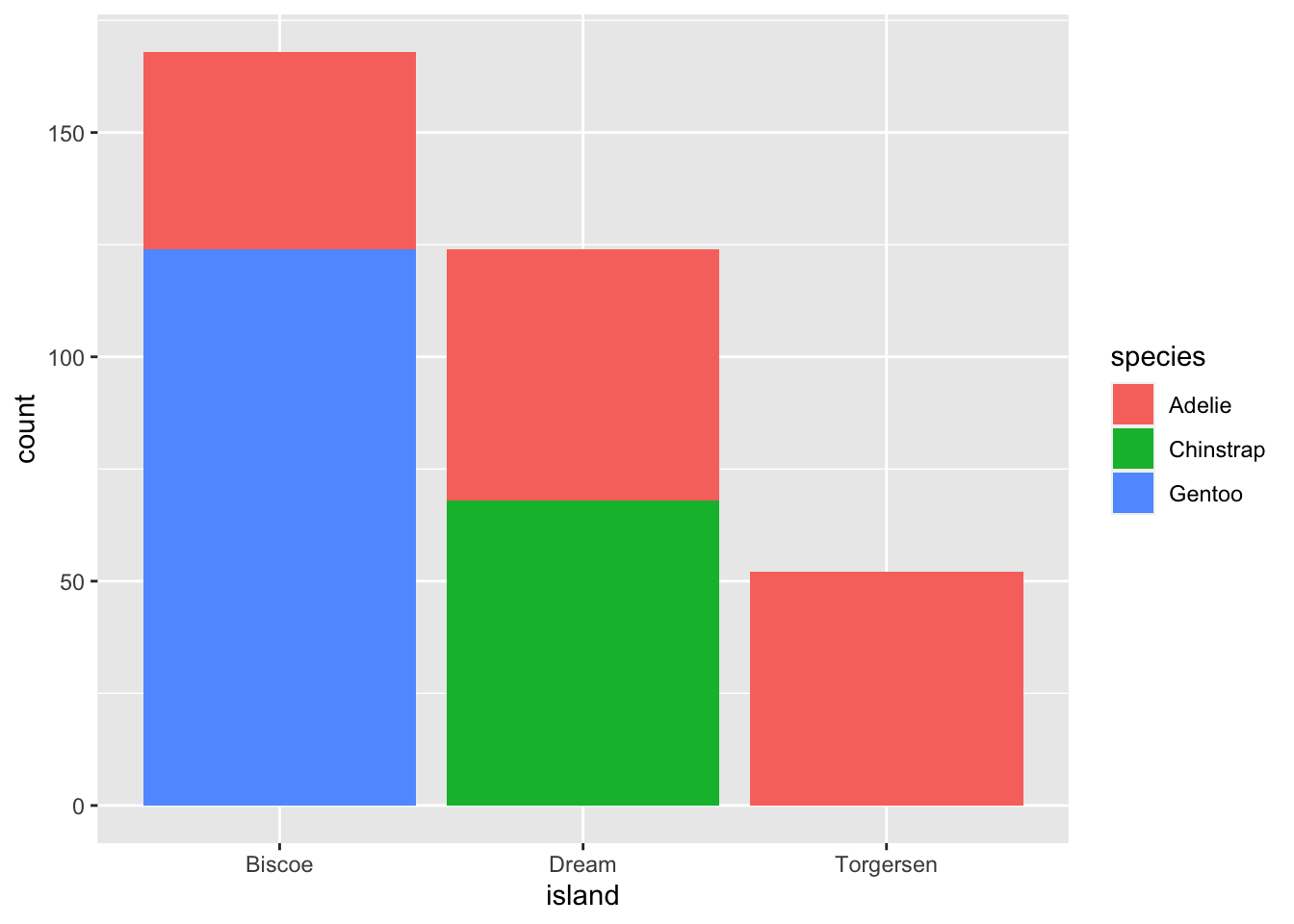This creates a stacked bar chart. These are generally more difficult to read than side-by-side bars. We can separate the portions of the stacked bar that correspond to each island and put them side-by-side by using the `position` argument for `geom_bar()` and setting it to “dodge”.

``````ggplot(data = penguins, aes(x = island)) +
geom_bar(aes(fill = species), position = "dodge")``````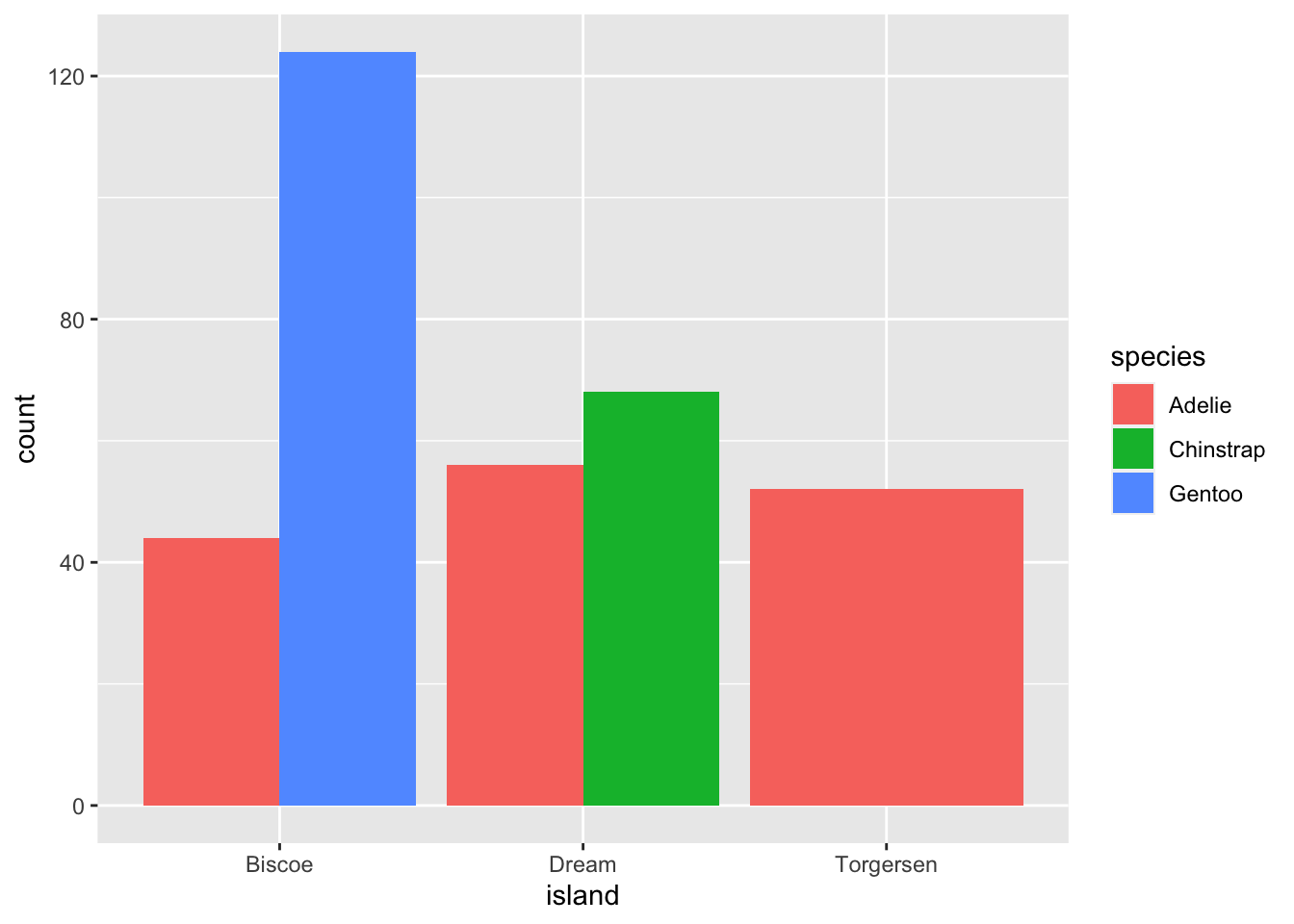## `ggplot2` themes

In addition to `theme_bw()`, which changes the plot background to white, `ggplot2` comes with several other themes which can be useful to quickly change the look of your visualization. The complete list of themes is available at http://docs.ggplot2.org/current/ggtheme.html. `theme_minimal()` and `theme_light()` are popular, and `theme_void()` can be useful as a starting point to create a new hand-crafted theme.

The ggthemes package provides a wide variety of options (including an Excel 2003 theme). The `ggplot2` extensions website provides a list of packages that extend the capabilities of `ggplot2`, including additional themes.

## Customization

Take a look at the `ggplot2` cheat sheet, and think of ways you could improve the plot.

## Exercise

With all of this information in hand, please take another five minutes to either improve one of the plots generated in this exercise or create a beautiful graph of your own.##### Kelsey E. Gonzalez, PhD
###### Sr Data Scientist

Data Scientist; Computational Social Scientist.